## 1. Overview

In this article, we're going to compare BitSets and boolean[] in terms of performance in different scenarios.

We usually use the term performance very loosely with different meanings in mind. Therefore, we'll start by looking at various definitions of the term “performance”.

Then, we're going to use two different performance metrics for benchmarks: memory footprint and throughput. To benchmark the throughput, we'll compare a few common operations on bit-vectors.

## 2. Definition of Performance

Performance is a very general term to refer to a wide range of “performance” related concepts!

Sometimes we use this term to talk about the startup speed of a particular application; that is, the amount of time the application takes before being able to respond to its first request.

In addition to startup speed, we may think about memory usage when we talk about performance. So the memory footprint is another aspect of this term.

It's possible to interpret the “performance” as how “fast” our code works. So the latency is yet another performance aspect.

For some applications, it's very critical to know the system capacity in terms of operations per second. So the throughput can be another aspect of performance.

Some applications only after responding to a few requests and getting “warmed up” technically speaking, can operate on their peak performance level. Therefore, time to peak performance is another aspect.

The list of possible definitions goes on and on! Throughout this article, however, we're going to focus on only two performance metrics: memory footprint and throughput.

## 3. Memory Footprint

Although we might expect booleans to consume just one bit, each boolean in a boolean[] consumes one byte of memory. This is mainly to avoid word tearing and accessibility issues. Therefore, if we need a vector of bits, boolean[] will have a pretty significant memory footprint.

To make matters more concrete, we can use Java Object Layout (JOL) to inspect the memory layout of a boolean[] with, say, 10,000 elements:

``````boolean[] ba = new boolean[10_000];
System.out.println(ClassLayout.parseInstance(ba).toPrintable());``````

This will print the memory layout:

``````[Z object internals:
OFFSET  SIZE      TYPE DESCRIPTION               VALUE
0     4           (object header)           01 00 00 00 (1)
4     4           (object header)           00 00 00 00 (0)
8     4           (object header)           05 00 00 f8 (-134217723)
12     4           (object header)           10 27 00 00 (10000)
16 10000   boolean [Z.                       N/A
Instance size: 10016 bytes``````

As shown above, this boolean[] consumes around 10 KB of memory.

On the other hand, BitSet is using a combination of primitive data types (specifically long) and bitwise operations to achieve one bit per flag footprint. So a BitSet with 10,000 bits will consume much less memory compared to a boolean[] with the same size:

``````BitSet bitSet = new BitSet(10_000);
System.out.println(GraphLayout.parseInstance(bitSet).toPrintable());``````

Similarly, this will print the memory layout of the BitSet:

``````[email protected] object externals:
ADDRESS       SIZE TYPE             PATH
76beb8190         24 java.util.BitSet
76beb81a8       1272 [J               .words   ``````

As expected, the BitSet with the same number of bits consumes around 1 KB, which is far less than the boolean[].

We can also compare the memory footprint for the different number of bits:

``````Path path = Paths.get("footprint.csv");
try (BufferedWriter stream = Files.newBufferedWriter(path, StandardOpenOption.CREATE)) {
stream.write("bits,bool,bitset\n");

for (int i = 0; i <= 10_000_000; i += 500) {
System.out.println("Number of bits => " + i);

boolean[] ba = new boolean[i];
BitSet bitSet = new BitSet(i);

long baSize = ClassLayout.parseInstance(ba).instanceSize();
long bitSetSize = GraphLayout.parseInstance(bitSet).totalSize();

stream.write((i + "," + baSize + "," + bitSetSize + "\n"));

if (i % 10_000 == 0) {
stream.flush();
}
}
}``````

The above code will compute the object size for both types of bit-vectors with different lengths. Then it writes and flushes the size comparisons to a CSV file.

Now if we plot this CSV file, we'll see that the absolute difference in memory footprint grows with the number of bits: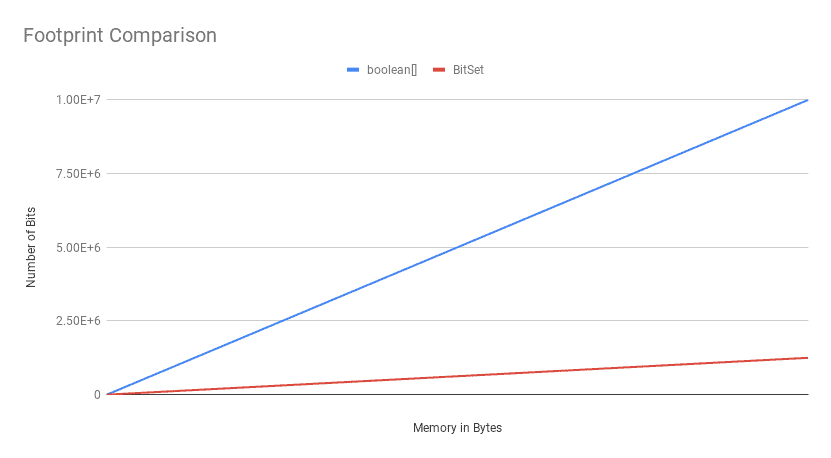The key takeaway here is, the BitSet beats the boolean[] in terms of the memory footprint, except for a minimal number of bits.

## 4. Throughput

To compare the throughput of BitSet and boolean[] with each other, we'll conduct three benchmarks based on three different and yet everyday operations on bit-vectors:

• Getting the value of a particular bit
• Setting or clearing the value of a specific bit
• Counting the number of set bits

This is the common setup we're going to use for the throughput comparison of bit-vectors with different lengths:

``````@State(Scope.Benchmark)
@BenchmarkMode(Mode.Throughput)
public class VectorOfBitsBenchmark {

private boolean[] array;
private BitSet bitSet;

@Param({"100", "1000", "5000", "50000", "100000", "1000000", "2000000", "3000000",
"5000000", "7000000", "10000000", "20000000", "30000000", "50000000", "70000000", "1000000000"})
public int size;

@Setup(Level.Trial)
public void setUp() {
array = new boolean[size];
for (int i = 0; i < array.length; i++) {
}

bitSet = new BitSet(size);
for (int i = 0; i < size; i++) {
}
}

// omitted benchmarks
}``````

As shown above, we're creating boolean[]s and BitSets with lengths in the 100-1,000,000,000 range. Also, after setting a few bits in the setup process, we'll perform different operations on both the boolean[] and BitSets.

### 4.1. Getting a Bit

At first glance, the direct memory access in boolean[] seems to be more efficient than performing two bitwise operations per get in BitSets (left-shift plus an and operation). On the other hand, memory compactness of BitSets may allow them to fit more values inside a cache line.

Let's see which one wins! Here are the benchmarks that JMH will run with a different value of the size state each time:

``````@Benchmark
public boolean getBoolArray() {
}

@Benchmark
public boolean getBitSet() {
}``````

### 4.2. Getting a Bit: Throughput

We're going to run the benchmarks using the following command:

``\$ java -jar jmh-1.0-SNAPSHOT.jar -f2 -t4 -prof perfnorm -rff get.csv getBitSet getBoolArray``

This will run the get-related benchmarks using four threads and two forks, profile their execution stats using the perf tool on Linux and outputs the result into the bench-get.csv file. The “-prof perfnorm” will profile the benchmark using the perf tool on Linux and normalizes the performance counters based on the number of operations.

Since the command result is so verbose, we're going only to plot them here. Before that, let's see the basic structure of each benchmark result:

``````"Benchmark","Mode","Threads","Samples","Score","Score Error (99.9%)","Unit","Param: size"
"getBitSet","thrpt",4,40,184790139.562014,2667066.521846,"ops/s",100
"getBitSet:L1-dcache-stores","thrpt",4,2,6.042285,NaN,"#/op",100
"getBitSet:branch-misses","thrpt",4,2,0.000451,NaN,"#/op",100
"getBitSet:branches","thrpt",4,2,12.985709,NaN,"#/op",100
"getBitSet:dTLB-store-misses","thrpt",4,2,0.000034,NaN,"#/op",100
"getBitSet:dTLB-stores","thrpt",4,2,6.035930,NaN,"#/op",100
"getBitSet:instructions","thrpt",4,2,90.781944,NaN,"#/op",100``````

As shown above, the result is a comma-separated list of fields each representing a metric. For instance, “thrpt” represents the throughput, “L1-dcache-load-misses” is the number of cache misses for the level 1 data cache, “L1-icache-load-misses” is the number of cache misses for the level 1 instruction cache, and “instructions” represents the number of CPU instructions for each benchmark. Also, the last field represents the number of bits, and the first one represents the benchmark method name.

This is how the benchmark results look like for throughput on a typical Digitial Ocean droplet with a 4-core Intel(R) Xeon(R) CPU 2.20GHz: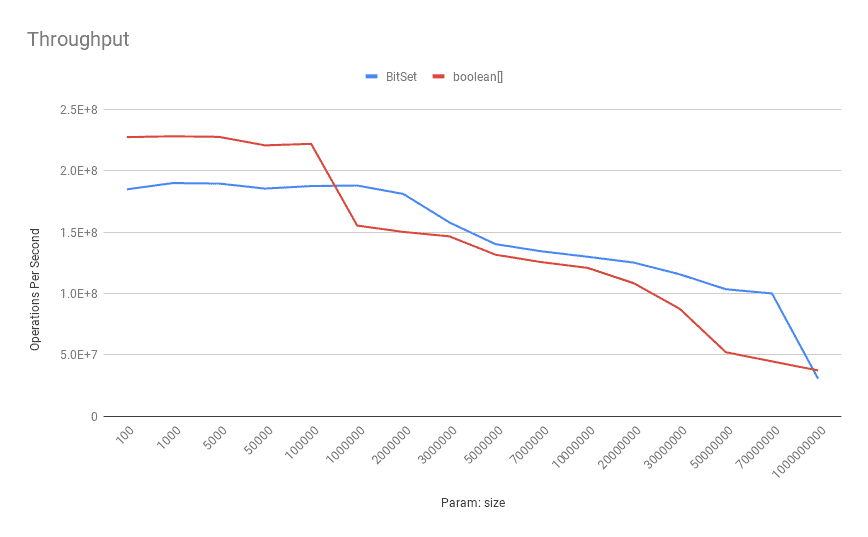As shown above, the boolean[] has a better throughput on smaller sizes. When the number of bits increases, the BitSet outperforms the boolean[] in terms of throughput. To be more specific, after 100,000 bits, the BitSet shows superior performance.

### 4.3. Getting a Bit: Instructions per Operation

As we expected, the get operation on a boolean[] has fewer instructions per operation: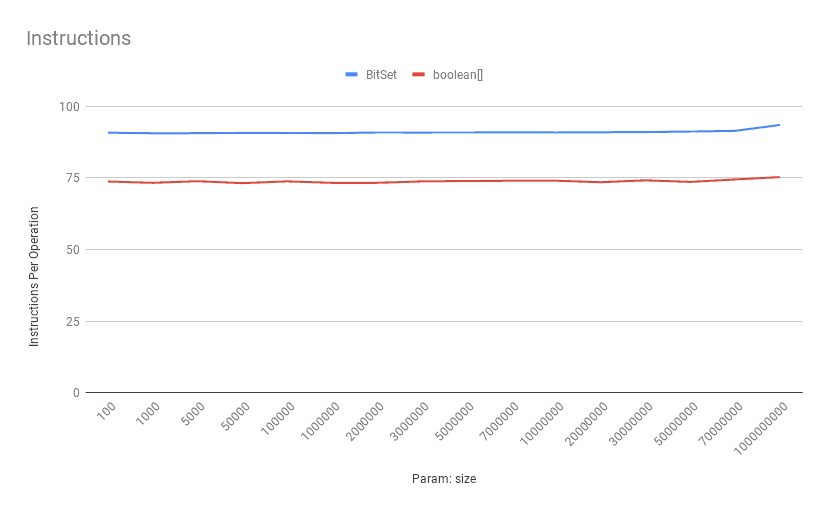### 4.4. Getting a Bit: Data Cache Misses

Now, let's see how data cache misses are looking for these bit-vectors: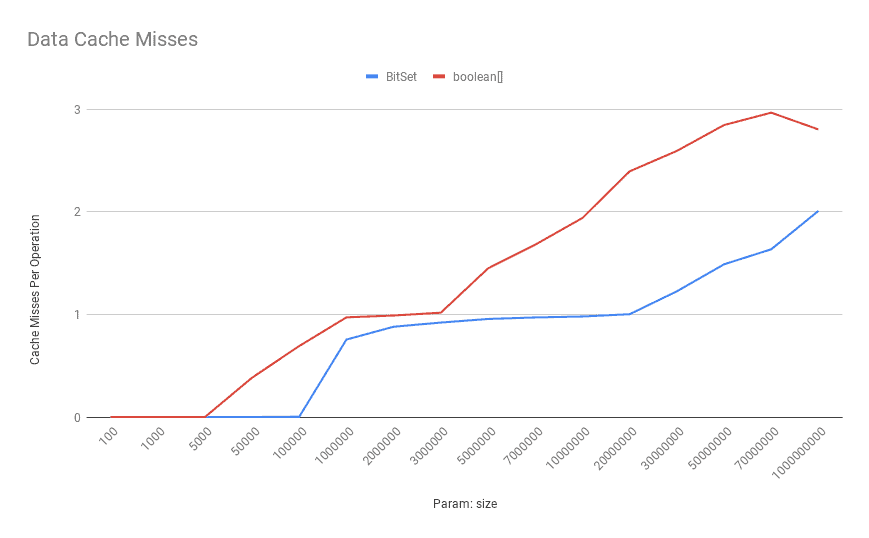As shown above, the number of data cache misses for the boolean[] increases as the number of bits goes up.

So cache misses are much more expensive than executing more instructions here. Therefore, the BitSet API outperforms the boolean[] in this scenario most of the time.

### 4.5. Setting a Bit

To compare the throughput of set operations, we're going to use these benchmarks:

``````@Benchmark
public void setBoolArray() {
int index = ThreadLocalRandom.current().nextInt(size);
array[index] = true;
}

@Benchmark
public void setBitSet() {
int index = ThreadLocalRandom.current().nextInt(size);
bitSet.set(index);
}``````

Basically, we're picking a random bit index and set it to true. Similarly, we can run these benchmarks using the following command:

``\$ java -jar jmh-1.0-SNAPSHOT.jar -f2 -t4 -prof perfnorm -rff set.csv setBitSet setBoolArray``

Let's see how the benchmark results look like for these operations in terms of throughput: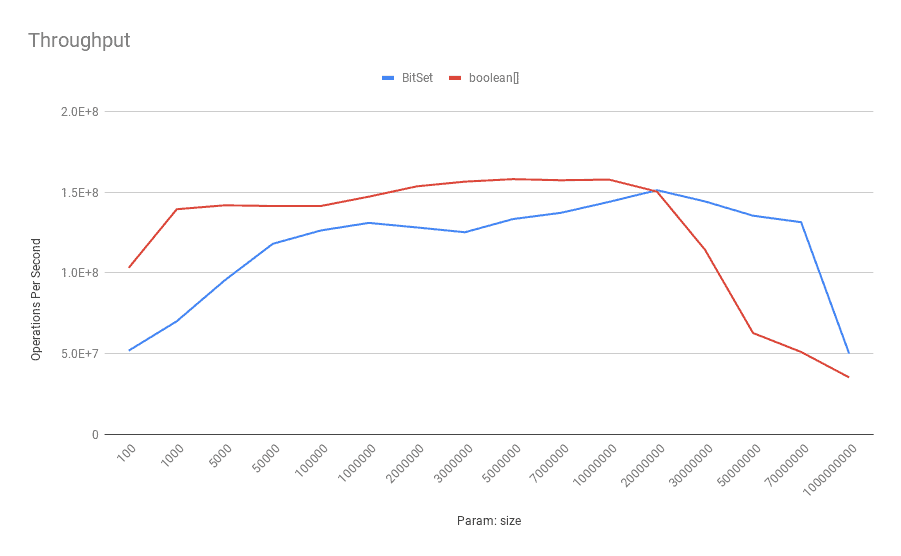This time the boolean[] outperforms the BitSet most of the time except for the very large sizes. Since we can have more BitSet bits inside a cache line, the effect of cache misses and false sharing can be more significant in BitSet instances.

Here is the data cache miss comparison: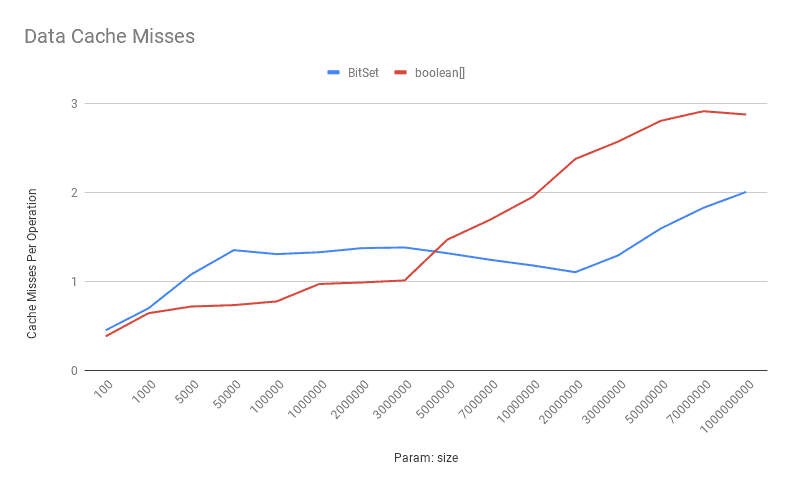As shown above, the data cache misses for boolean[] is pretty low for low to a moderate number of bits. Again, when the number of bits increases, the boolean[] encounters more cache misses.

Similarly, the instructions per operation for boolean[] is reasonably less than the BitSet: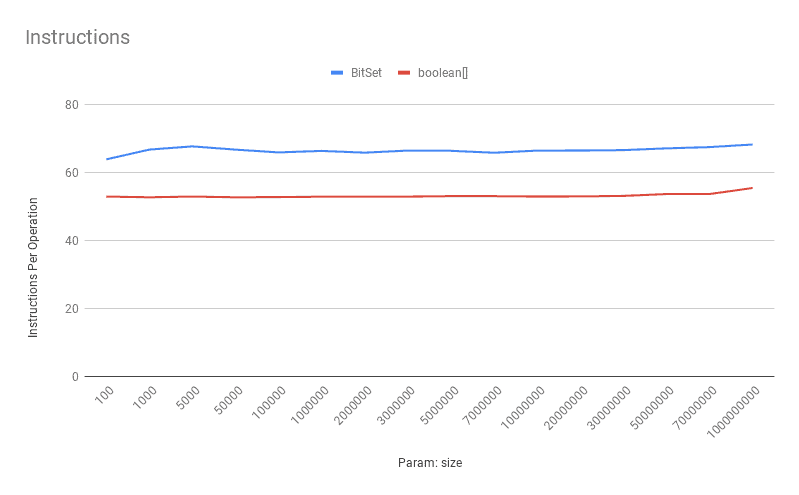### 4.6. Cardinality

One of the other common operations in such bit-vectors is to count the number of set-bits. This time we're going to run these benchmarks:

``````@Benchmark
public int cardinalityBoolArray() {
int sum = 0;
for (boolean b : array) {
if (b) sum++;
}

return sum;
}

@Benchmark
public int cardinalityBitSet() {
return bitSet.cardinality();
}``````

Again we can run these benchmarks with the following command:

``\$ java -jar jmh-1.0-SNAPSHOT.jar -f2 -t4 -prof perfnorm -rff cardinal.csv cardinalityBitSet cardinalityBoolArray``

Here's what the throughput looks like for these benchmarks: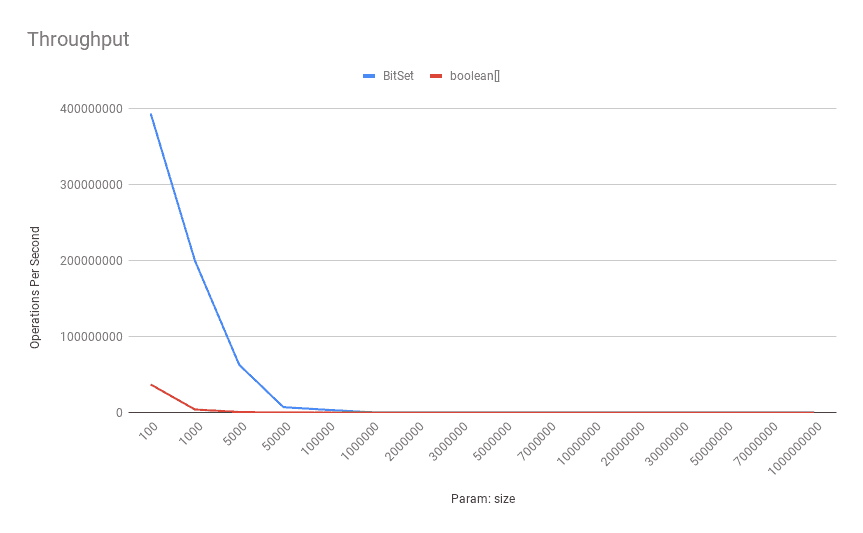In terms of cardinality throughput, the BitSet API outperforms the boolean[] almost all the time because it has much fewer iterations. To be more specific, the BitSet only has to iterate its internal long[] which has much less number of elements compared to the corresponding boolean[].

Also, because of this line and random distribution of set-bits in our bit-vectors:

``````if (b) {
sum++;
}``````

The cost of branch misprediction can be decisive, too: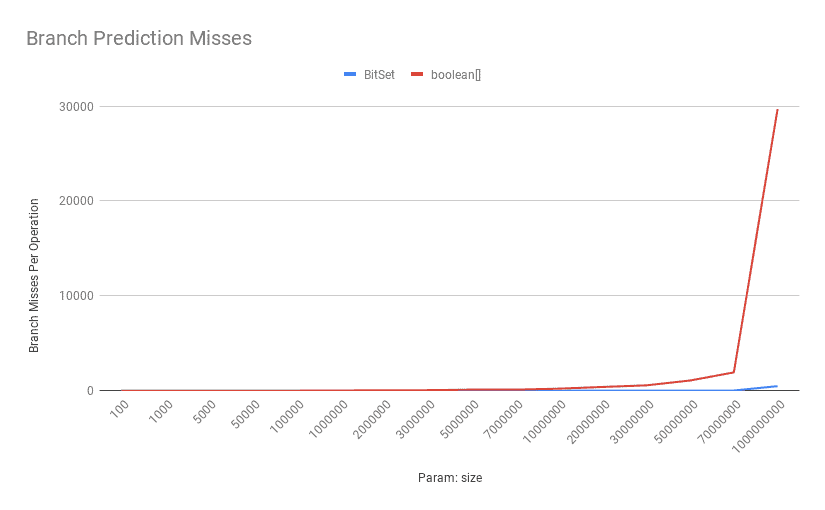As shown above, as the number of bits increases, the number of mispredictions for the boolean[] goes up significantly.

## 5. Conclusion

In this article, we compared the throughput of BitSet and boolean[] in terms of three common operations: getting a bit, setting a bit, and calculating cardinality. In addition to throughput, we saw that the BitSet uses much less memory compared to a boolean[] with the same size.

To recap, in single-bit read-heavy scenarios, the boolean[] outperforms the BitSet in smaller sizes. However, when the number of bits increases, the BitSet has superior throughput.

Moreover, in single-bit write-heavy scenarios, the boolean[] exhibits a superior throughput almost all the time except for a very large number of bits. Also, in the batch read scenarios, the BitSet API completely dominates the boolean[] approach.

We used the JMH-perf integration to capture low-level CPU metrics such as L1 Data Cache Misses or Missed Branch Predictions. As of Linux 2.6.31, perf is the standard Linux profiler capable of exposing useful Performance Monitoring Counters or PMCs. It's also possible to use this tool separately. To see some examples of this standalone usage, it's highly recommended to read Branden Greg‘s blog.

As usual, all the examples are available over on GitHub. Moreover, the CSV results of all conducted benchmarks are also accessible on GitHub.

### Get started with Spring 5 and Spring Boot 2, through the Learn Spring course:

>> CHECK OUT THE COURSE